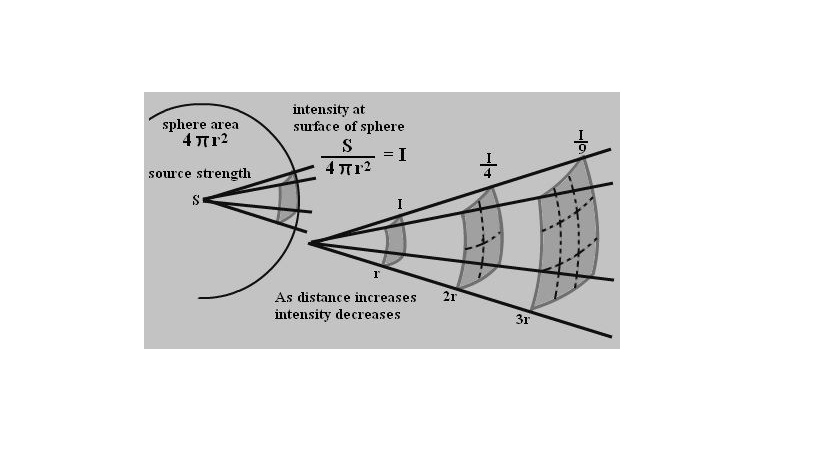# Inverse Square Law

In physics, there are many laws that define objects, light, Earth and many more. These laws are formed with respect to some fundamental quantity. Snell’s law, laws of reflection, laws of refraction are some of the laws which are formed on the nature of light. We know that light travels in a straight line and can undergo various phenomenon like diffraction, polarisation, interference, reflection and refraction too.

In this article, let us study a law that defines the intensity of light with respect to distance. Let us learn about the inverse square law.

## Inverse Square Law

This law explains the strength of light with respect to the distance of the source.

Inverse square law states that “the Intensity of the radiation is inversely proportional to the square of the distance”.

Or

“The intensity of the light to an observer from a source is inversely proportional to the square of the distance from the observer to the source”.

As per this law, light loses its brightness or luminosity as it moves away from the source. For example: when you switch on the light in one corner of the room and when you move away from the source, the light appears dim or less bright due to an increase in the distance (away from the source).

The below figure explains the source of light and the source distance relationship.Consider the distance as 2, and when the distance is squared, it results in 4. The inverse of 4 would be ¼. So when we compare, we can note that the resultant is quarter the original power.

Hence light intensity is 1/d^2

If the distance between an object and the light source is doubled, a given area experiences one-fourth part of the light from the source. When the distance from the source is tripled, the given area experiences one-ninth part of the light.

## The Formula of Inverse-Square Law

The formula of inverse-square law is given as,
$$I\alpha\frac{1}{d^{2}}$$

Where,

d is the distance

Consider light sources of intensity I1 and I2 at the distances d1 and d2. The inverse-square law is articulated as:
$$\frac{I_{1}}{I_{2}}\alpha \frac{d_{2}^{2}}{d_{1}^{2}}$$

Where the intensity of light is measured by candela or Lumen, and the distance is measured in meters.

## Applications Of Inverse Square Law

• This law is used to calculate the intensity of any given radiation or distance.
• Inverse-square law helps to calculate the source to film distances in X-ray techniques.
• It also helps to determine the time of x-ray exposure and the intensity of the x-ray tube used in the process.
• Using the standard candle approach, when the brightness of the source is known, it helps to calculate the distance from the Earth.
• Inverse-square law is used to measure various astronomical distances.

 Related links Relative motion Protection against earthquake Lenz law Radioactivity – alpha decay

## Frequently Asked Questions – FAQs

### What is the unit to measure the intensity of light?

Lumen or candela.

Refractometer.

24m.

### What is the inverse-square law formula?

$$I\alpha\frac{1}{d^{2}}$$

### What happens to the brightness of light when moved away from the source?

The brightness decreases.

Stay tuned with BYJU’S for more such interesting articles on physics, chemistry and maths in an engaging way with video explanations.